#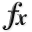N-D Test Functions J¶

class go_benchmark.JennrichSampson(dimensions=2)

Jennrich-Sampson test objective function.

This class defines the Jennrich-Sampson global optimization problem. This is a multimodal minimization problem defined as follows: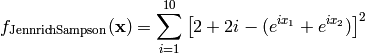Here,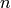represents the number of dimensions and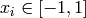for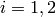.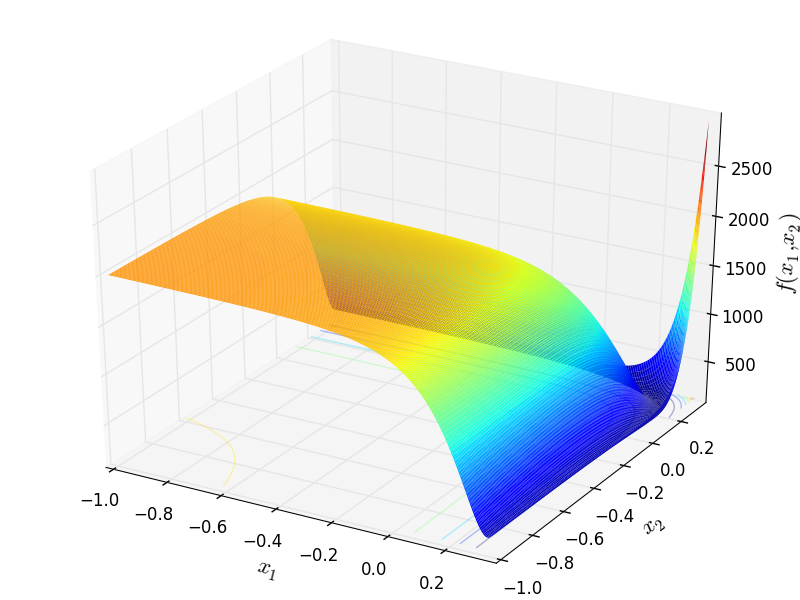Two-dimensional Jennrich-Sampson function

Global optimum: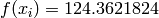for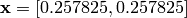.

class go_benchmark.Judge(dimensions=2)

Judge test objective function.

This class defines the Judge global optimization problem. This is a multimodal minimization problem defined as follows: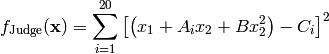Where, in this exercise: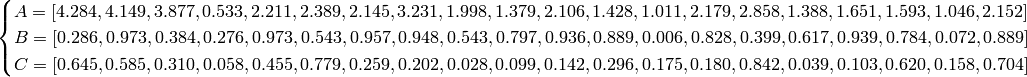Here,represents the number of dimensions andfor.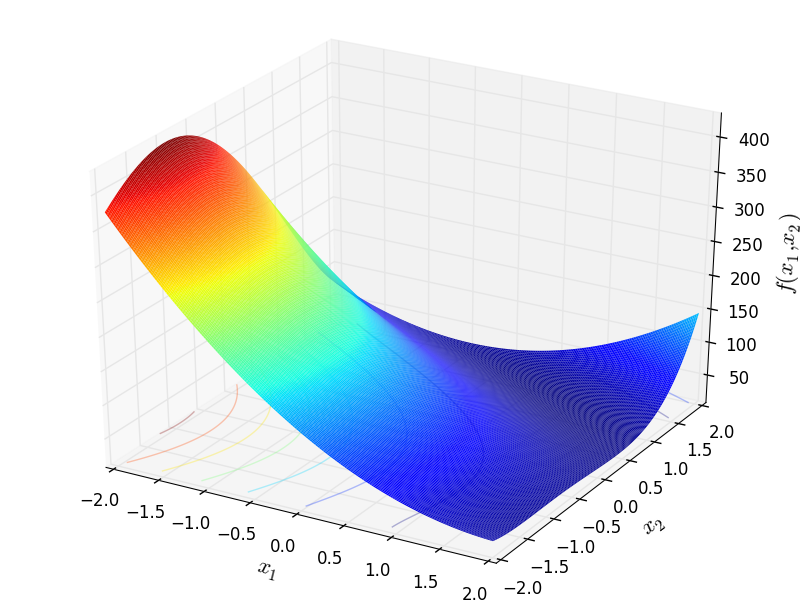Two-dimensional Judge function

Global optimum: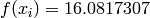for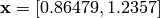.

#### Previous topic

N-D Test Functions I

#### Next topic

N-D Test Functions K